• TensorFlow手写数字识别预处理代码，备注详细可以直接运行，为后续具体算法的 实现提供了可靠、规范的数据源。处理内容包括MNIST数据的下载、读取、整形、归一化、标签独热编码以及画图显示等。
• tensorflow手写数字识别python源码官网案例，带详细注释，适合刚初学tensorflow的mnist数据集训练识别， 相关链接： 手写数字识别 ----在已经训练好的数据上根据28*28的图片获取识别概率（基于Tensorflow,Python） ...
• 采用tensorflow深度学习框架对手写数字识别的一整套代码
• 用python编写的，基于tensorflow深度学习的框架的手写数字识别的完整代码，有注释，可运行，无bug
• 使用TensorFlow实现简单的手写数字0-9识别，下载后解压配置环境运行。
• 带界面的手写数字识别程序，Qt开发界面，使用python基于TensorFlow设计手写数字识别算法，并编程实现GUI界面，构建手写数字识别系统。内附有相关开发文档。
• 深度学习入门视频-唐宇迪 （笔记加自我整理） 深度学习 第三章 tensorflow基本操作 1.tensorflow常见操作 2.tensorflow实现线性回归 3.tensoflow实现手写数字识别

深度学习入门视频-唐宇迪 （笔记加自我整理）

深度学习 第三章 tensorflow手写数字识别

1.tensorflow常见操作

这里使用的是tensorflow1.x版本，tensorflow基本构成单位是张量tensor，区别在于不同阶。零阶张量，也叫标量，就是一个数值；一阶张量，可以理解为一维向量，比如列向量或者行向量；二阶张量，可以理解为二维矩阵；还可以有多阶张量，最简单的区分方法是看中括号"["，有多少个中括号，是几阶的张量。

tensorflow也有固定的语法套路，有三步，第一步定义变量或者常量，第二步初始化参数，第三步把定义的变量放到session中真正执行起来。session可以理解为一个容器，使用sess.run() 真正执行代码，得到结果。

• session定义

输入的数据有两种，一种是变量，定义方式是tf.Variable()， 一种是常量，tf.constant(). 定义输入之后，tf.global_variable_initializer()表示初始化所有定义的变量和常量，一定要有这一步。然后采用with tf.Session()的结构，定义一个session，把所有数据放入sess.run()中，执行输出。

变量和常量赋值有两种方式，一种是定义的时候直接复制，一种是定义的时候先占位置，使用的时候再赋值。下面这种方式是声明的变量的时候直接给值，类似于到图书馆直接找位置坐下来看书。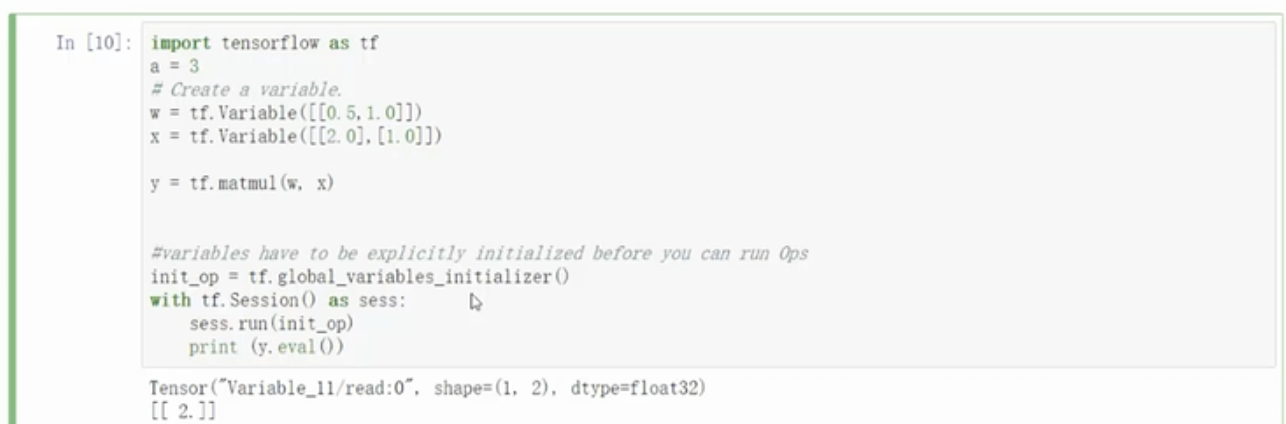还有一种方式类似延迟加载，就是定义变量或者常量的时候，先不执行具体数值，而是先指定输入的类型，在真正执行代码的时候，把变量对应真正的值传入再进行计算。关键组合是placeholder（占位）+ feed_dict={}。类似于去图书馆的占座，需要先想清楚占几个位置，但是具体是谁坐哪个位置，要等到人来之后才能定。

placeholder用来占位置，feed_dict={}格式需要是字典的形式，把变量和对应值传入到容器中，执行得到具体结果。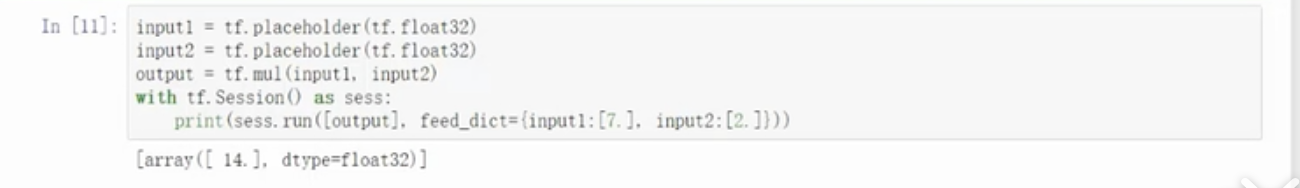• 常用操作

tensorflow有大量对矩阵的运算，通常会涉及到矩阵的初始化，一般有三种方式，第一种所有数据全赋值为0，第二种所有数据全赋值为1，第三种所有数据根据正态分布随机生成数值。初始化的时候需要执行要生成矩阵的维度，矩阵中数据的类型不是必须的，如果不传入则使用默认值。一般矩阵数值类型范围主要是，整型，浮点型，布尔型，字符串类型。

•
• zeros ：
• x1= tf.zeros([3,4], dtype=tf.int32)  ,
• 生成3行4列的二阶张量，类型为int32，值全部为0
• ones：
• x2 = tf.ones([3,4], dtype=tf.int32),
• 生成3行4列的二阶张量，类型为int32，值全部为1
• random_normal:
• x3 = tf.random_normal([2,3], mean=0,stddev=4),
• 生成2行3列的二阶张量，这里默认类型为float32，这里生成的生态分布式均值为0，标准差为4的随机数据。
• shuffle：
• x4 = tf.random_shuffle(c) , 对数据进行打乱操作，
• 一般在切分训练集和测试集的时候，先打乱再切分，比较常用。
• conver_to_tensor：
• a = numpy.zeros((3,3))
• ta = tf.conver_to_tensor(a)，将numpy数组转换成tensor张量
• 一定要注意，tenforflow中可以用于计算结构是张量，其他都不可以用于计算，但是numpy数组和tensor张量有很多操作相似，可以进行类型转换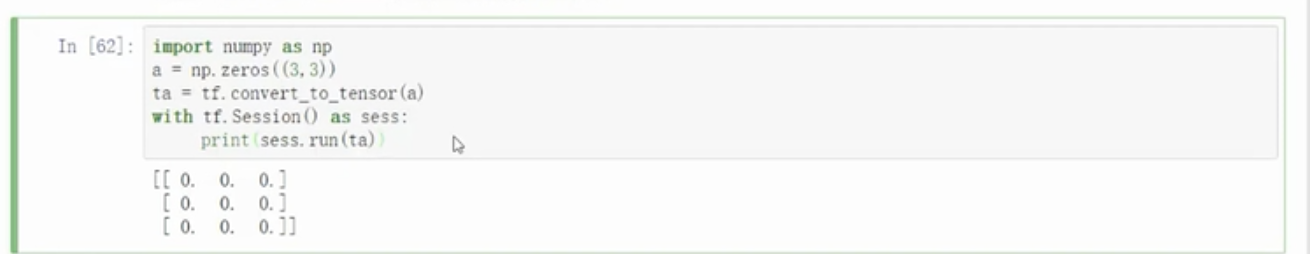2.tenforflow实现线性回归

使用tensorflow实现线性回归，为简单起见，随机构造这一组数据使其符合线性关系y=w*x+b，这里x只有一维，即一个特征。构造输入数据后，要构造训练代码。首先初始化参数，这里权重W矩阵，采用random_uniform生成一维矩阵，数值介于[-1,1]之间的随机数；偏置项B矩阵，初始化为全0的一维矩阵。定义得分函数y，定义损失函数loss，这里采用均方误差作为优化目标，采用随机梯度下降法来优化损失函数，让loss朝最小值方向更新，最后每20次训练打印一次损失值loss，直到执行完迭代次数，损失值收敛。

• 训练代码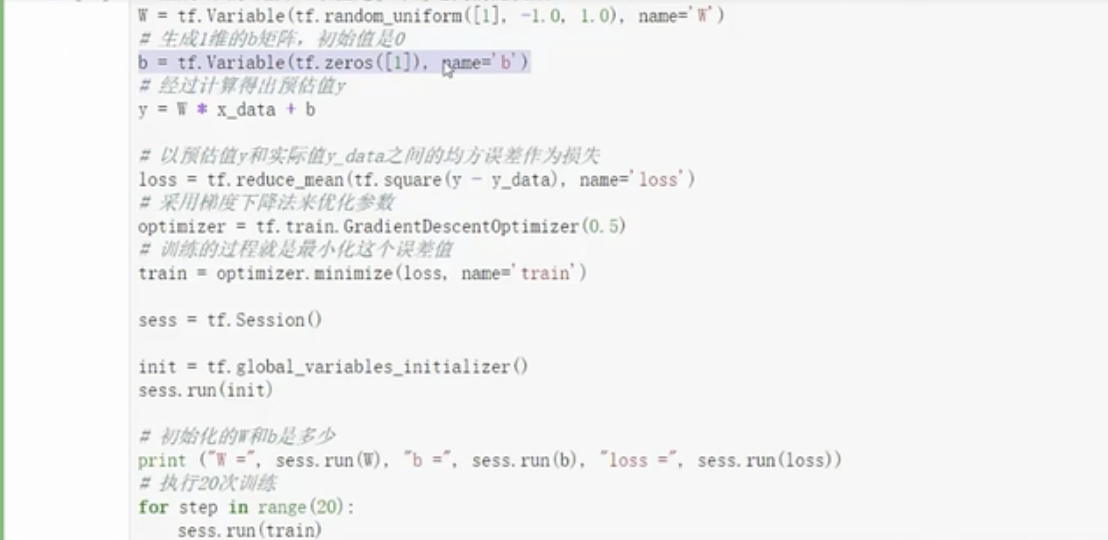3.手写字体识别

一般神经网络多分类任务通常采用softmax分类器，对应的10个类别需要编码转换成计算机识别的方式，这里采用one-hot编码方式。one-hot也叫独热编码，是对于离散类别数据常用的编码方式，这里一共有10个类别，用10个数字表示，每个位置上的数字代表一个类别，比如数字图片类别是0，那么代表0类别的对应位置上的数值为1，其他类别对应位置都为0，所以叫独热编码，可以理解为只有一个类别是热的，其他的都是凉的。比如这里数字0可以表示为[ 1, 0, 0, 0, 0, 0, 0, 0, 0, 0] ， 数字7可以表示为 [ 0, 0, 0, 0, 0, 0, 0, 1, 0, 0]，其中一张图片大概是这样子。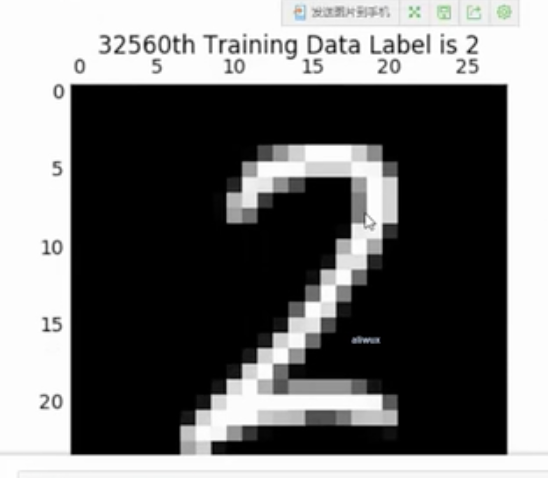接下来需要定义神经网络的层次，以及各参数的维度大小。通常情况下，神经网络模型很少自己定义层次，比如需要几层网络，每一层有多少个神经元，一般都是采用现成的模型，一般是于论文证明并且工业界使用效果不错的模型。这里是为了实现手写识别的例子，注意实际中很少采用自定义模型即可。

这里涉及采用4层模型，输入层是32*32的图片，可以采用批处理方式一次输入多张图片，但是要指定每次使用几张图片，图片大小和批处理的个数是固定不能改变的。中间隐含层设定为2层，隐含层1的神经元采用256个，隐含层2的神经元采用128个，最后的输出层采用softmax分类器，这里的类别是10种，所以输出类别为10。 下面就开始计算矩阵参数的大小了。输入层到隐含层1中间的权重参数W1 = 784* 256， 隐含层1到隐含层2的权重参数W2= 256*128， 隐含层2到输出层的权重参数W3 = 128* 10， 还要注意每个权重矩阵都要对应一个偏置项。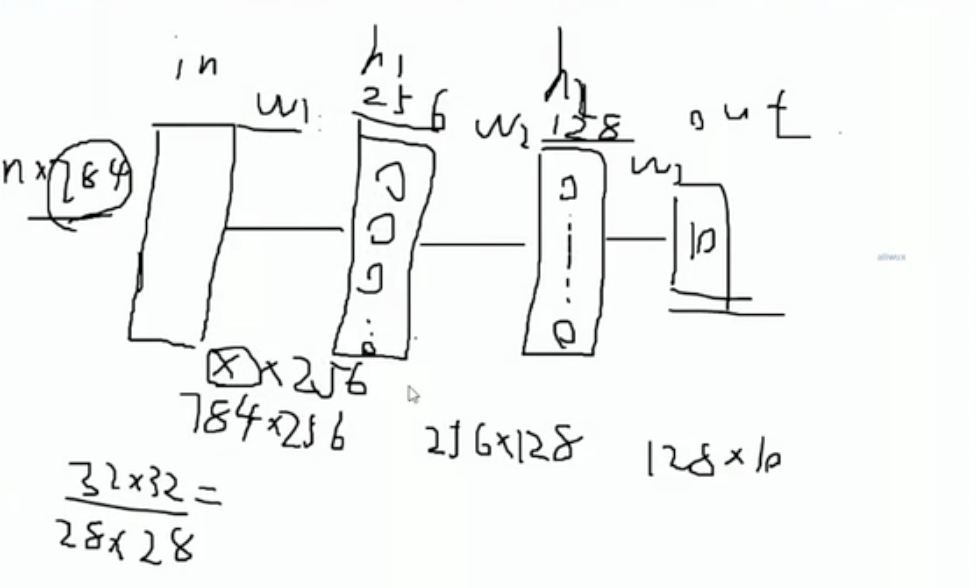大体代码如下：

•       定义各参数，执行维度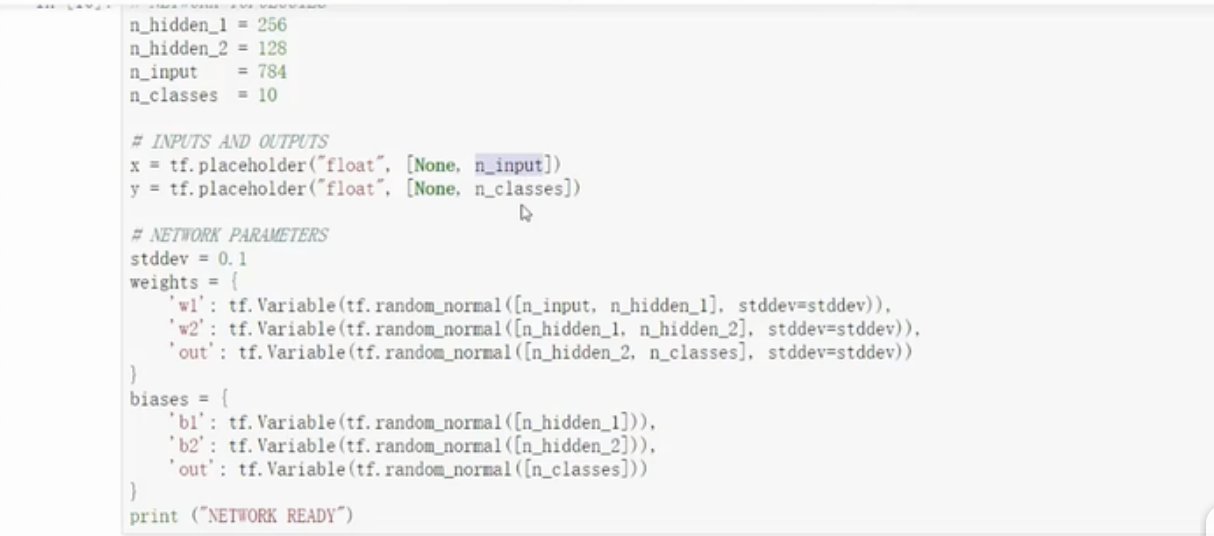• 定义两个隐含层，这里采用sigmoid作为激活函数，用于去线性化。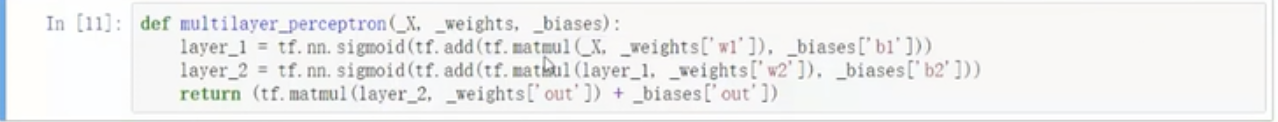• 定义程序主代码，即得分函数，损失函数，优化方式，评估方式。并初始代码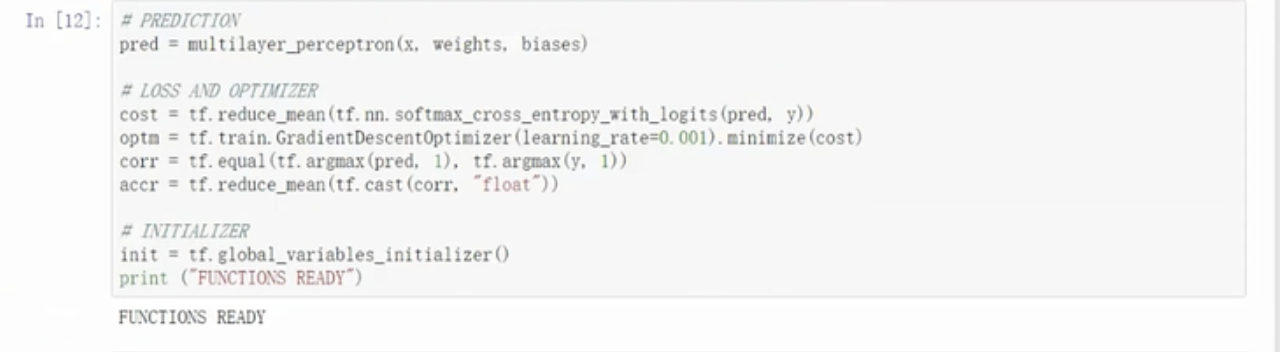• 执行程序，并设定中间打印训练集结果和测试集结果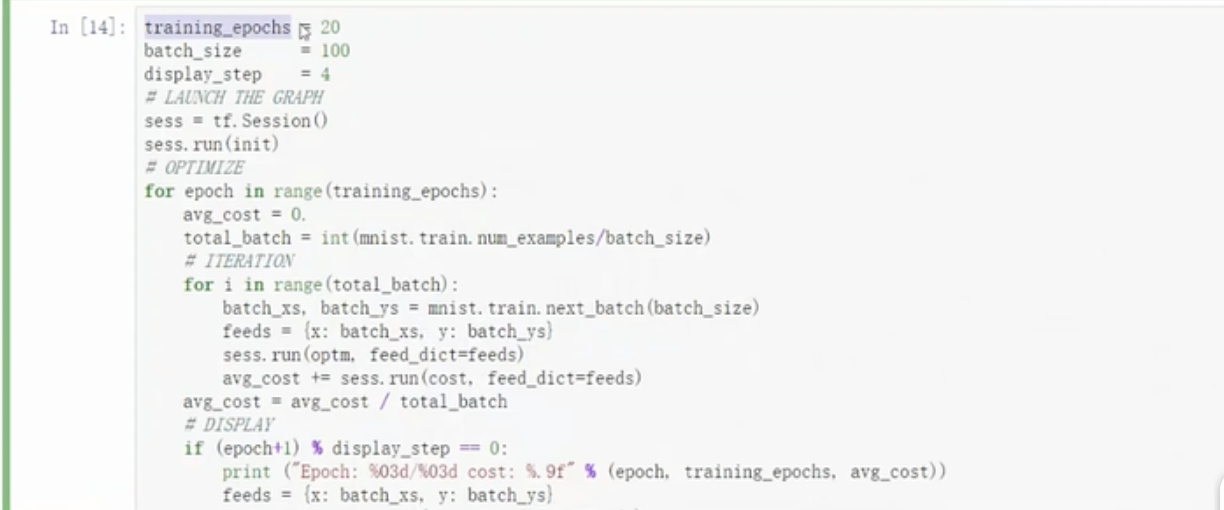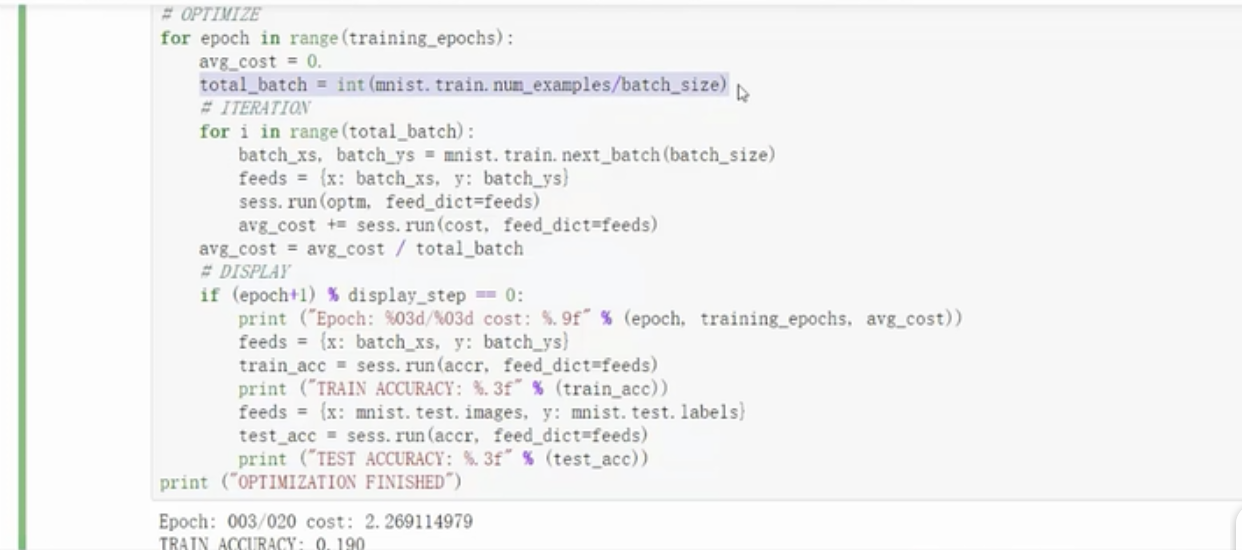• 打印结果如下：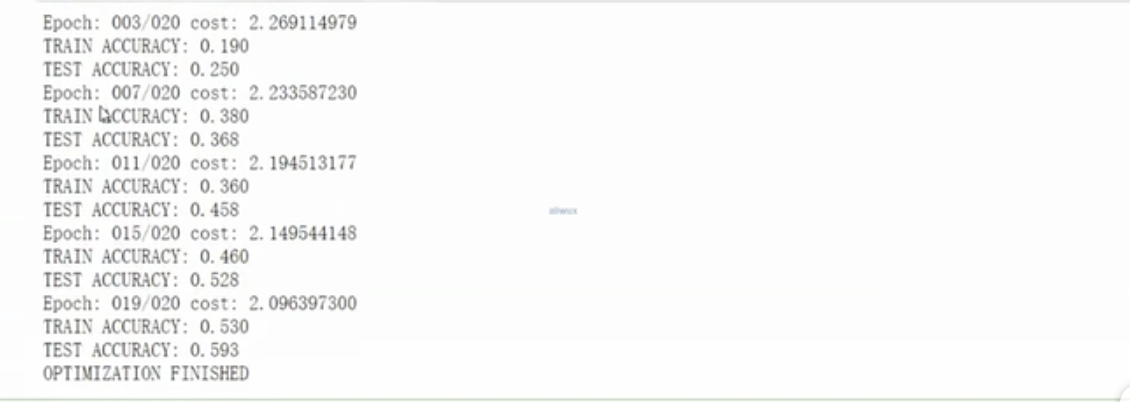展开全文深度学习 tensorflow
• 基于python3.7版本的tensorflow2.0实现mnist手写数字识别代码
• 初次接触TensorFlow，而手写数字训练识别是其最基本的入门教程，网上关于训练的教程很多，但是模型的测试大多都是官方提供的一些素材，能不能自己随便写一串数字让机器识别出来呢？纸上得来终觉浅，带着这个疑问昨晚...

初次接触TensorFlow，而手写数字训练识别是其最基本的入门教程，网上关于训练的教程很多，但是模型的测试大多都是官方提供的一些素材，能不能自己随便写一串数字让机器识别出来呢？纸上得来终觉浅，带着这个疑问昨晚研究了下，利用这篇文章来记录下自己的一些心得！

以下这个图片是我随机写的一串数字，我的目标是利用训练好的模型来识别出图片里面的手写数字，开始实战！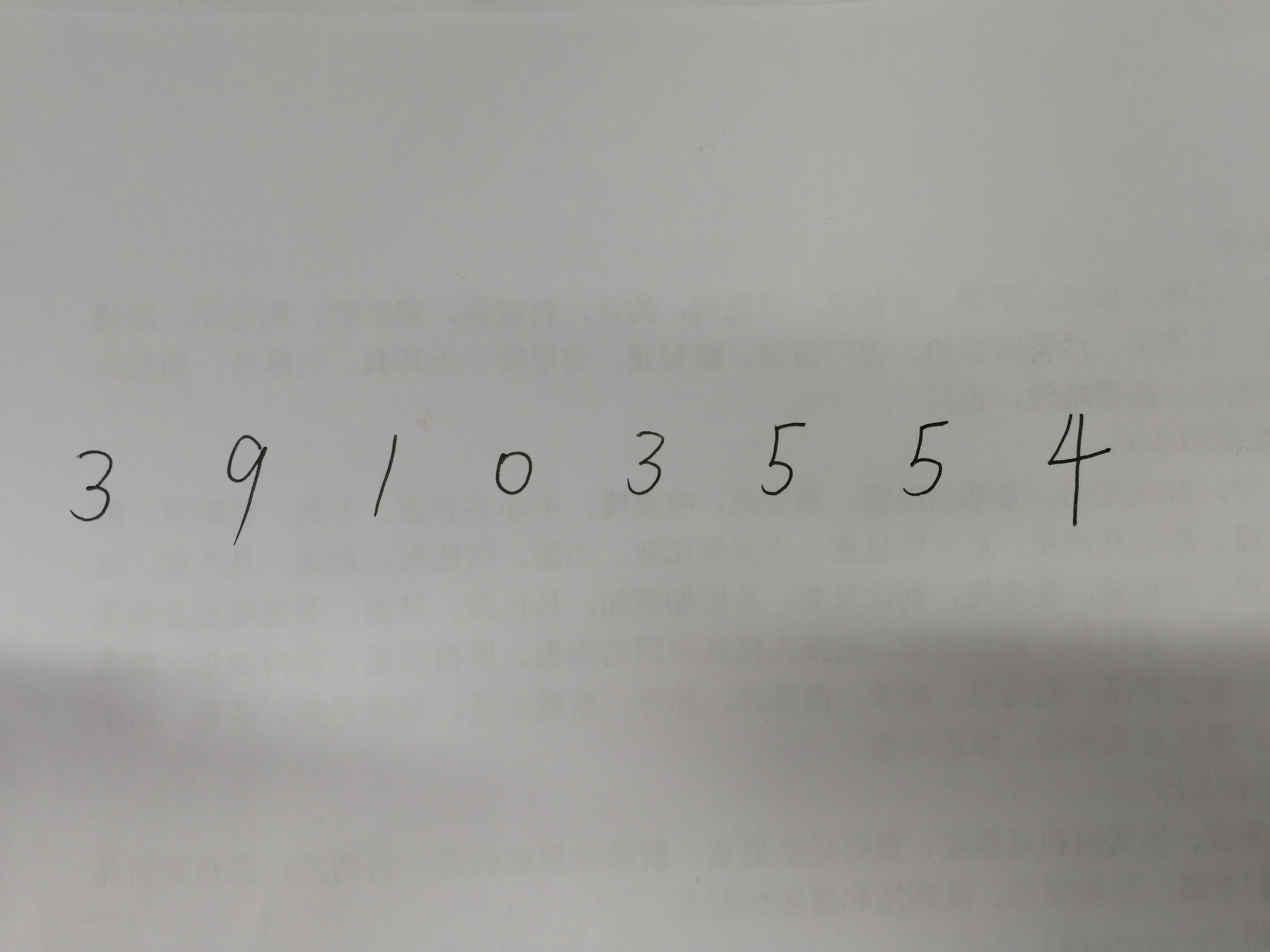2层卷积神经网络的训练：

from tensorflow.examples.tutorials.mnist import input_data
# 保存模型需要的库
from tensorflow.python.framework.graph_util import convert_variables_to_constants
from tensorflow.python.framework import graph_util
# 导入其他库
import tensorflow as tf
import cv2
import numpy as np
# 获取MINIST数据
# 创建会话
sess = tf.InteractiveSession()
# 占位符
x = tf.placeholder("float", shape=[None, 784], name="Mul")
y_ = tf.placeholder("float", shape=[None, 10], name="y_")
# 变量
W = tf.Variable(tf.zeros([784, 10]), name='x')
b = tf.Variable(tf.zeros(), 'y_')
# 权重
def weight_variable(shape):
initial = tf.truncated_normal(shape, stddev=0.1)
return tf.Variable(initial)
# 偏差
def bias_variable(shape):
initial = tf.constant(0.1, shape=shape)
return tf.Variable(initial)
# 卷积
def conv2d(x, W):
return tf.nn.conv2d(x, W, strides=[1, 1, 1, 1], padding='SAME')
# 最大池化
def max_pool_2x2(x):
return tf.nn.max_pool(x, ksize=[1, 2, 2, 1],

# 相关变量的创建
W_conv1 = weight_variable([5, 5, 1, 32])
b_conv1 = bias_variable()
x_image = tf.reshape(x, [-1, 28, 28, 1])
h_conv1 = tf.nn.relu(conv2d(x_image, W_conv1) + b_conv1)
h_pool1 = max_pool_2x2(h_conv1)
W_conv2 = weight_variable([5, 5, 32, 64])
b_conv2 = bias_variable()
# 激活函数
h_conv2 = tf.nn.relu(conv2d(h_pool1, W_conv2) + b_conv2)
h_pool2 = max_pool_2x2(h_conv2)
W_fc1 = weight_variable([7 * 7 * 64, 1024])
b_fc1 = bias_variable()
W_fc2 = weight_variable([1024, 10])
b_fc2 = bias_variable()
h_pool2_flat = tf.reshape(h_pool2, [-1, 7 * 7 * 64])
h_fc1 = tf.nn.relu(tf.matmul(h_pool2_flat, W_fc1) + b_fc1)
keep_prob = tf.placeholder("float", name='rob')
h_fc1_drop = tf.nn.dropout(h_fc1, keep_prob)
# 用于训练用的softmax函数
y_conv = tf.nn.softmax(tf.matmul(h_fc1_drop, W_fc2) + b_fc2, name='res')
# 用于训练作完后，作测试用的softmax函数
y_conv2 = tf.nn.softmax(tf.matmul(h_fc1, W_fc2) + b_fc2, name="final_result")
# 交叉熵的计算，返回包含了损失值的Tensor。
cross_entropy = -tf.reduce_sum(y_ * tf.log(y_conv))
# 优化器，负责最小化交叉熵

correct_prediction = tf.equal(tf.argmax(y_conv, 1), tf.argmax(y_, 1))
# 计算准确率
accuracy = tf.reduce_mean(tf.cast(correct_prediction, "float"))
# 初始化所以变量
sess.run(tf.global_variables_initializer())
# 保存输入输出，可以为之后用
# 训练开始
for i in range(10000):
batch = mnist.train.next_batch(50)
if i % 100 == 0:
train_accuracy = accuracy.eval(feed_dict={
x: batch, y_: batch, keep_prob: 1.0})
print("step %d, training accuracy %g" % (i, train_accuracy))
# run()可以看做输入相关值给到函数中的占位符，然后计算的出结果，这里将batch，给xbatch给y_
train_step.run(feed_dict={x: batch, y_: batch, keep_prob: 0.5})
# 将当前图设置为默认图
graph_def = tf.get_default_graph().as_graph_def()
# 将上面的变量转化成常量，保存模型为pb模型时需要,注意这里的final_result和前面的y_con2是同名，只有这样才会保存它，否则会报错，
# 如果需要保存其他tensor只需要让tensor的名字和这里保持一直即可
output_graph_def = tf.graph_util.convert_variables_to_constants(sess,graph_def, ['final_result'])
# 用saver 保存模型
saver = tf.train.Saver()
saver.save(sess, "model_data/model")

网络训练成功后在model_data文件夹里有如下四个文件：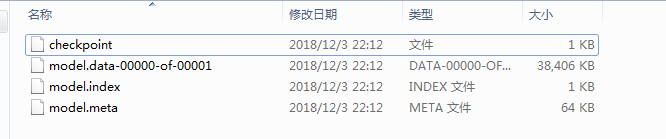网络模型的验证可大致从以下三个部分来进行：

3. 降采样为28*28的大小来进行识别。

代码部分如下所示：

"""
基于TensorFlow的手写数字识别
Author_Zjh
2018/12/3
"""
import numpy as np
import cv2
import matplotlib.pyplot as plt
import imutils
import matplotlib.patches as mpatches
from skimage import data,segmentation,measure,morphology,color
import tensorflow as tf
class Number_recognition():
""" 模型恢复初始化"""
def __init__(self,img):
self.sess = tf.InteractiveSession()
saver = tf.train.import_meta_graph('model_data/model.meta')
saver.restore(self.sess, 'model_data/model') #模型恢复
# graph = tf.get_default_graph()
# 获取输入tensor,,获取输出tensor
self.input_x = self.sess.graph.get_tensor_by_name("Mul:0")
self.y_conv2 = self.sess.graph.get_tensor_by_name("final_result:0")
self.Preprocessing(img)#图像预处理
def recognition(self,im):
im = cv2.resize(im, (28, 28), interpolation=cv2.INTER_CUBIC)
x_img = np.reshape(im, [-1, 784])
output = self.sess.run(self.y_conv2, feed_dict={self.input_x: x_img})
print('您输入的数字是 %d' % (np.argmax(output)))
return np.argmax(output)#返回识别的结果

def Preprocessing(self,image):
if image.shape>800:
image = imutils.resize(image, height=800) #如果图像太大局部阈值分割速度会稍慢些，因此图像太大时进行降采样

img = cv2.cvtColor(image, cv2.COLOR_BGR2GRAY)  # convert to gray picture
m1, n1 = img.shape
k = int(m1 / 19) + 1
l = int(n1 / 19) + 1
img = cv2.GaussianBlur(img, (3, 3), 0)  # 高斯滤波
imm = img.copy()
# 基于Niblack的局部阈值分割法，对于提取文本类图像分割效果比较好
for x in range(k):
for y in range(l):
s = imm[19 * x:19 * (x + 1), 19 * y:19 * (y + 1)]
me = s.mean()  # 均值
var = np.std(s)  # 方差
t = me * (1 - 0.2 * ((125 - var) / 125))
ret, imm[19 * x:19 * (x + 1), 19 * y:19 * (y + 1)] = cv2.threshold(
imm[19 * x:19 * (x + 1), 19 * y:19 * (y + 1)], t, 255, cv2.THRESH_BINARY_INV)
label_image = measure.label(imm)  # 连通区域标记
for region in measure.regionprops(label_image):  # 循环得到每一个连通区域属性集
# 忽略小区域
if region.area < 100:
continue
minr, minc, maxr, maxc = region.bbox# 得到外包矩形参数
cv2.rectangle(image, (minc, minr), (maxc, maxr), (0, 255, 0), 2)#绘制连通区域
im2 = imm[minr - 5:maxr + 5, minc - 5:maxc + 5] #获得感兴趣区域，也即每个数字的区域
number = self.recognition(im2)#进行识别
cv2.putText(image, str(number), (minc, minr - 10), 0, 2, (0, 0, 255), 2)#将识别结果写在原图上
cv2.imshow("Nizi", imm)
cv2.imshow("Annie", image)
cv2.waitKey(0)
if __name__=='__main__':
x=Number_recognition(img)

分割结果如下所示：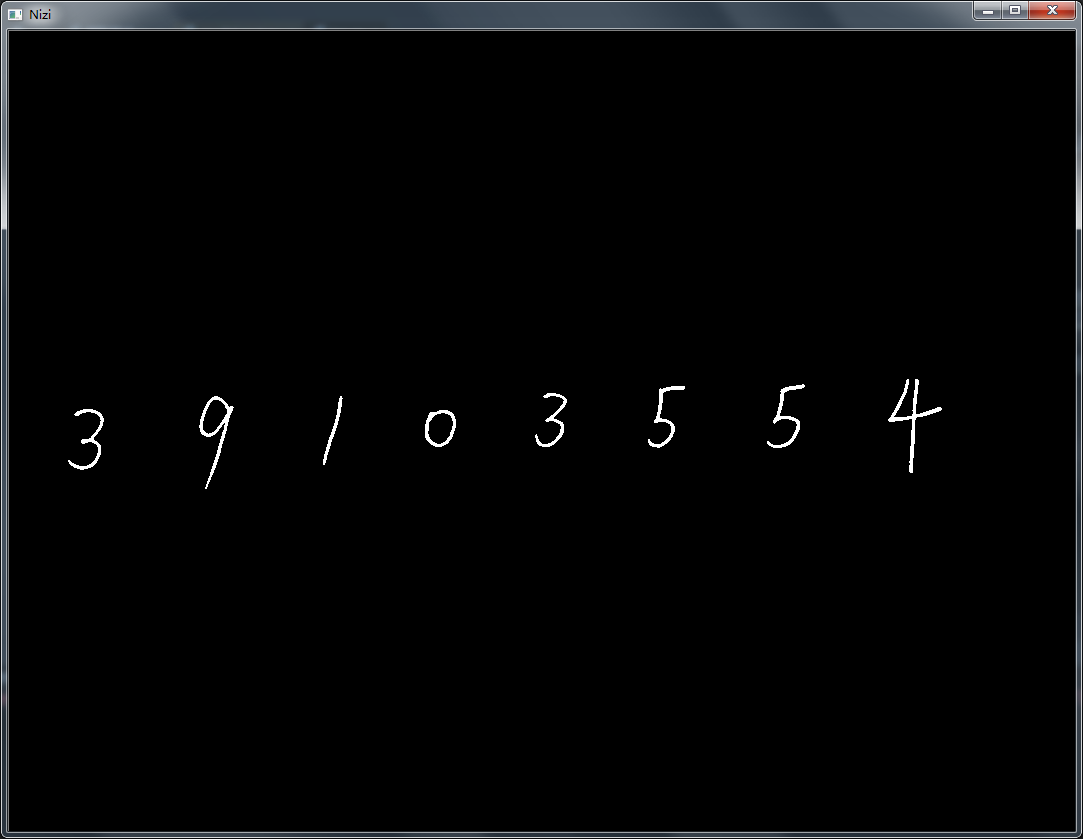识别结果如下所示：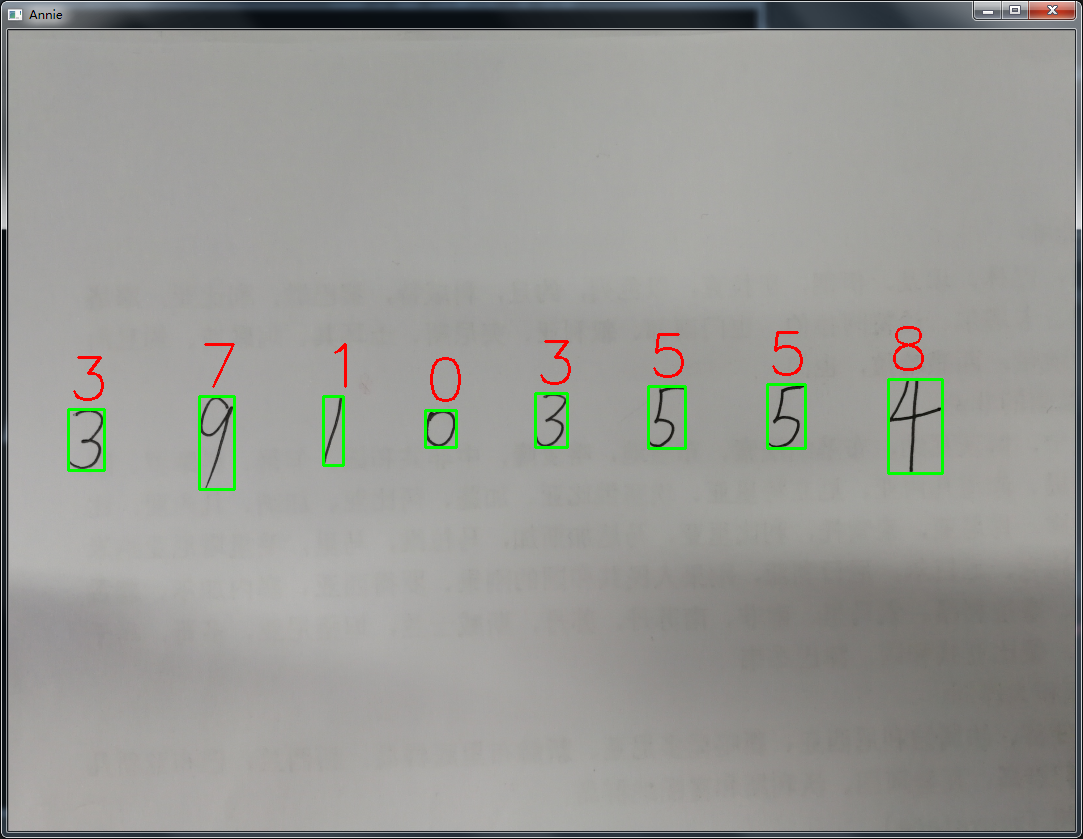发现9和4识别错误，其余的均识别正确，有可能是数据量和网络迭代次数较少的原因！

展开全文• 基础知识 本节内容可以跳过直接看后面的处理 MNIST数据集 MNIST数据集的官网是Yann LeCun’s website。可以使用下面的python代码自动下载数据集。...from tensorflow.examples.tutorials.mnist import input_d...

一、基础知识

基础知识可以跳过，可以直接看后面的代码实现

MNIST数据集

MNIST数据集的官网是Yann LeCun’s website。可以使用下面的python代码自动下载数据集。

#已经下载input_data.py
#import input_data
#没有下载input_data.py
from tensorflow.examples.tutorials.mnist import input_data

下载下来的数据集被分成两部分：60000行的训练数据集（mnist.train）和10000行的测试数据集（mnist.test）。这样的切分很重要，在机器学习模型设计时必须有一个单独的测试数据集不用于训练而是用来评估这个模型的性能，从而更加容易把设计的模型推广到其他数据集上（泛化）。
每一个MNIST数据单元有两部分组成：一张包含手写数字的图片和一个对应的标签。我们把这些图片设为“xs”，把这些标签设为“ys”。训练数据集和测试数据集都包含xs和ys，比如训练数据集的图片是 mnist.train.images ，训练数据集的标签是 mnist.train.labels。

每一张图片包含28像素X28像素。我们可以用一个数字数组来表示这张图片：我们把这个数组展开成一个向量，长度是 28x28 = 784。如何展开这个数组（数字间的顺序）不重要，只要保持各个图片采用相同的方式展开。从这个角度来看，MNIST数据集的图片就是在784维向量空间里面的点, 并且拥有比较复杂的结构 (提醒: 此类数据的可视化是计算密集型的)。

展平图片的数字数组会丢失图片的二维结构信息。这显然是不理想的，最优秀的计算机视觉方法会挖掘并利用这些结构信息，我们会在后续教程中介绍。但是在这个教程中我们忽略这些结构，所介绍的简单数学模型，softmax回归(softmax regression)，不会利用这些结构信息。

一般的计算机视觉会先把图像转为图像矩阵，然后再转化为一维向量，处理图片过程如下：

from PIL import Image
#打开图像
image = Image.open("smallpi.jpg")
#转化成灰度图像,这一步不是必须的，但为了效率一般都会处理
image_gray = image.convert("L")
#Image对象转化成图像矩阵
image_array = np.array(image_gray )
#flatten可以将矩阵转化成一维序列
image_vectors = image_array.flatten()
#归一化处理到0-1范围内
image_vectors_float = image_vectors/255

因此，在MNIST训练数据集中，mnist.train.images 是一个形状为 [60000, 784] 的张量，第一个维度数字用来索引图片，第二个维度数字用来索引每张图片中的像素点。在此张量里的每一个元素，都表示某张图片里的某个像素的强度值，值介于0和1之间。相对应的MNIST数据集的标签是介于0到9的数字，用来描述给定图片里表示的数字。为了用于这个教程，我们使标签数据是"one-hot vectors"。 一个one-hot向量除了某一位的数字是1以外其余各维度数字都是0。所以在此教程中，数字n将表示成一个只有在第n维度（从0开始）数字为1的10维向量。比如，标签0将表示成([1,0,0,0,0,0,0,0,0,0,0])。因此， mnist.train.labels 是一个 [60000, 10] 的数字矩阵。我们可以通过下面的python代码查看mnist这个数据集的情况：

print(mnist.train.images.shape, mnist.train.labels.shape)
print(mnist.test.images.shape, mnist.test.labels.shape)
print(mnist.validation.images.shape, mnist.validation.labels.shape)

其中mnist.train.images返回训练集的图片信息，minist.test返回测试集的图片信息，mnist.validation.images返回测试集的图片信息。而labels就是图片标签：0-9shape就是矩阵数据的维度，比如 [[2,3],[3,5],[1,5]]通过shape运算就返回（3,2）。
以上运行结果为：

(55000, 784) (55000, 10)
(10000, 784) (10000, 10)
(5000, 784) (5000, 10)

Softmax回归

softmax用于多分类过程中，它将多个神经元的输出，映射到（0,1）区间内，可以看成概率来理解，从而来进行多分类！
假设我们有一个数组，V，Vi表示V中的第i个元素，那么这个元素的softmax值就是
s i = e i ∑ j e j s_i = \dfrac{e^i}{\sum_j e^j}
更形象的如下图表示：计算过程如下(用了四舍五入，保留2位有效数字)：
y 1 = e z 1 ∑ j = 1 3 e z j = e z 1 e z 1 + e z 2 + e z 3 y_1=\dfrac{e^{z_1}}{\sum_{j=1}^3e^{z_j}}=\dfrac{e^{z_1}}{e^{z_1}+e^{z_2}+e^{z_3}} = e 3 e 3 + e 1 + e − 3 = 20 20 + 2.7 + 0.05 = 0.88 =\dfrac{e^3}{e^3+e^1+e^{-3}} =\dfrac{20}{20+2.7+0.05}=0.88
y 2 = e z 2 ∑ j = 1 3 e z j = e z 2 e z 1 + e z 2 + e z 3 y_2=\dfrac{e^{z_2}}{\sum_{j=1}^3e^{z_j}}=\dfrac{e^{z_2}}{e^{z_1}+e^{z_2}+e^{z_3}} = e 1 e 3 + e 1 + e − 3 = 2.7 20 + 2.7 + 0.05 = 0.12 =\dfrac{e^1}{e^3+e^1+e^{-3}} =\dfrac{2.7}{20+2.7+0.05}=0.12
y 3 = e z 3 ∑ j = 1 3 e z j = e z 3 e z 1 + e z 2 + e z 3 y_3=\dfrac{e^{z_3}}{\sum_{j=1}^3e^{z_j}}=\dfrac{e^{z_3}}{e^{z_1}+e^{z_2}+e^{z_3}} = e − 3 e 3 + e 1 + e − 3 = 0.05 20 + 2.7 + 0.05 = 0 =\dfrac{e^{-3}}{e^3+e^1+e^{-3}} =\dfrac{0.05}{20+2.7+0.05}=0
其中：
z 1 = w 11 × x 1 + w 21 × x 2 + w 31 × x 3 z_1=w_{11} \times x_1+w_{21} \times x_2+w_{31} \times x_3
z 2 = w 12 × x 1 + w 22 × x 2 + w 32 × x 3 z_2=w_{12} \times x_1+w_{22} \times x_2+w_{32} \times x_3
z 3 = w 13 × x 1 + w 23 × x 2 + w 33 × x 3 z_3=w_{13} \times x_1+w_{23} \times x_2+w_{33} \times x_3
softmax直白来说就是将原来输出是3,1,-3通过softmax函数一作用，就映射成为(0,1)的值，而这些值的累和为1（满足概率的性质），那么我们就可以将它理解成概率，在最后选取输出结点的时候，我们就可以选取概率最大（也就是值对应最大的）结点，作为我们的预测目标！

对于softmax回归模型可以用下面的图解释，对于输入的xs加权求和，再分别加上一个偏置量，最后再输入到softmax函数中：如果把它写成一个等式，我们可以得到：我们也可以用向量表示这个计算过程：用矩阵乘法和向量相加。这有助于提高计算效率。（也是一种更有效的思考方式）更进一步，可以写成更加紧凑的方式：
y = s o f t m a x ( W x + b ) y=softmax(Wx+b)

二、使用 TensorFlow 实现Softmax回归模型

1、定义x,y两个Placeholder,所谓Placehoder,初学者可以理解为一种容器，后面我们将会把上面的训练集数据mnist.train.images及minst.train.labels输入到x,y中。
我们希望能够输入任意数量的MNIST图像，每一张图展平成784维的向量。我们用2维的浮点数张量来表示这些图，这个张量的形状是[None，784 ]。（这里的None表示此张量的第一个维度可以是任何长度的。）

import tensorflow as tf

#定义两个placeholder
x = tf.placeholder(tf.float32,[None,784])
y = tf.placeholder(tf.float32,[None,10])

这里的参数x将会是输入的图片数据即为[None,784],None就是图片的数量，因为我们训练的时候并不是一张一张图片输入的，因为一张一张输入的效率低，而且会因为一张噪音图片的对整个网络造成巨大影响，正确的训练方法应该是将100张图片组成的数据集[100,784]输入神经网络进行训练（当然，这里的100也可以是其他数值）。然后得到神经网络的输出prediction,这里的prediction就是标签y的预测值，也是一个10维的向量，例如[0.12,0.22,0.21,0.86,0.33,0.01,0.81,0.15,0.54,0.13]

2、接下来我们定义权重值和偏置量。

W = tf.Variable(tf.zeros([784,10]))
b = tf.Variable(tf.zeros())

在这里，我们都用全为零的张量来初始化W和b。因为我们要学习W和b的值，它们的初值可以随意设置。一般的情况下我们都是采用随机设置。

3、创建模型。

prediction = tf.nn.softmax(tf.matmul(x,W)+b)

用tf.matmul(​​X，W)表示矩阵 x x 乘以矩阵 W W ，对应之前等式里面的 W x Wx ，这里x是一个2维张量拥有多个输入。然后再加上矩阵 b b ，把和输入到tf.nn.softmax函数里面。

4、定义损失函数(也叫成本函数)和优化算法。
这里定义二次损失函数和梯度优化算法，我们的目的就是通过更新神经网络参数W,b来让loss的最小，而更新 W W , b b 的操作就是train_step.

#二次代价函数
loss = tf.reduce_mean(tf.square(y-prediction))
#使用梯度下降法

其中二次代价函数(也称为方差)为
l o s s = 1 2 N ∑ n = 1 N ( y − p r e d i c t i o n ) 2 loss = \dfrac{1}{2N}\sum_{n=1}^N(y - prediction)^2
从sigmoid函数的图像就会发现，它的两侧几乎就是平的，导致它的方差在大部分情况下很小，这样在训练参数的时候收敛地就会很慢，交叉熵就是用来解决这个问题的。它的定义如下：
H y ′ ( y ) = − ∑ i y i ′ l o g ( y i ) H_{y&#x27;}(y)=-\sum_iy&#x27;_ilog(y_i)
其中，y 是我们预测的概率分布, y’ 是实际的分布。
所以损失函数如下：
l o s s = − ∑ y l o g ( p r e d i c t i o n ) loss = -\sum ylog(prediction )
代码如下：

loss = -tf.reduce_sum(y*tf.log(prediction))

5、计算准确率
这里的tf.argmax(y,1)，tf.argmax(prediction,1)就是计算10维向量y及prediction中元素的最大值，也就是说预测的标签和实际标签相等时tf.equal返回真，否则返回假。最终预测正确的信息被保存到correct_prediction布尔型列表中，在通过tf.cast转化为float型，然后通过tf.reduce_mean求平均求出准确率。

#结果存放在一个布尔型列表中
correct_prediction = tf.equal(tf.argmax(y,1),tf.argmax(prediction,1))#argmax返回一维张量中最大的值所在的位置
#求准确率
accuracy = tf.reduce_mean(tf.cast(correct_prediction,tf.float32))

6、接着就进入正式训练了
首先通过init初始化变量，接着for循环训练40个回合。batch_size为每个批次的大小，n_batch为批次数。在每次训练一个回合后计算出测试集的准确率acc,并输出。

# 每个批次的大小
batch_size = 100
# 计算一共有多少个批次
n_batch = mnist.train.num_examples // batch_size

with tf.Session() as sess:
init = tf.global_variables_initializer()
sess.run(init)
for epoch in range(40):
for batch in range(n_batch):
batch_xs, batch_ys = mnist.train.next_batch(batch_size)
sess.run(train_step, feed_dict={x: batch_xs, y: batch_ys})

acc = sess.run(accuracy, feed_dict={x: mnist.test.images, y: mnist.test.labels})
print("Iter " + str(epoch) + ",Testing Accuracy " + str(acc))

Iter 0,Testing Accuracy 0.8335
Iter 1,Testing Accuracy 0.8706
Iter 2,Testing Accuracy 0.8815
Iter 3,Testing Accuracy 0.889
Iter 4,Testing Accuracy 0.8936
Iter 5,Testing Accuracy 0.8968
Iter 6,Testing Accuracy 0.8994
Iter 7,Testing Accuracy 0.9019
Iter 8,Testing Accuracy 0.9022
Iter 9,Testing Accuracy 0.9052
Iter 10,Testing Accuracy 0.9066

以上是程序的运行的结果，可以看到最终准确率为91.94%，这并不是很高的准确率，但却是一个入门的经典例子，后面我们将会讲到如何提高准确率.

全部代码为：

#coding: utf-8
#载入数据集
from tensorflow.examples.tutorials.mnist import input_data

print(mnist.train.images.shape, mnist.train.labels.shape)
print(mnist.test.images.shape, mnist.test.labels.shape)
print(mnist.validation.images.shape, mnist.validation.labels.shape)

import tensorflow as tf

#定义两个placeholder
x = tf.placeholder(tf.float32, [None, 784])
y = tf.placeholder(tf.float32, [None, 10])

#创建一个简单的神经网络
W = tf.Variable(tf.zeros([784, 10]))
b = tf.Variable(tf.zeros())
prediction = tf.nn.softmax(tf.matmul(x, W) + b)

#二次代价函数
loss = tf.reduce_mean(tf.square(y - prediction))
#使用梯度下降法

#结果存放在一个布尔型列表中
correct_prediction = tf.equal(tf.argmax(y,1),tf.argmax(prediction,1))#argmax返回一维张量中最大的值所在的位置
#求准确率
accuracy = tf.reduce_mean(tf.cast(correct_prediction,tf.float32))
#每个批次的大小
batch_size = 100
#计算一共有多少个批次
n_batch = mnist.train.num_examples // batch_size

with tf.Session() as sess:
init = tf.global_variables_initializer()
sess.run(init)
for epoch in range(40):
for batch in range(n_batch):
batch_xs, batch_ys = mnist.train.next_batch(batch_size)
sess.run(train_step, feed_dict={x: batch_xs, y: batch_ys})

acc = sess.run(accuracy, feed_dict={x: mnist.test.images, y: mnist.test.labels})
print("Iter " + str(epoch) + ",Testing Accuracy " + str(acc))

展开全文• import tensorflow as tf from tensorflow.examples.tutorials.mnist import input_data # 定义一个初始化权重的函数 def weight_variables(shape): w = tf.Variable(tf.random_normal(shape=shape, mean=...

1.黑马课程案例

import tensorflow as tf
from tensorflow.examples.tutorials.mnist import input_data

# 定义一个初始化权重的函数
def weight_variables(shape):
w = tf.Variable(tf.random_normal(shape=shape, mean=0.0, stddev=1.0))#返回随机正态分布的随机值
return w

# 定义一个初始化偏置的函数
def bias_variables(shape):
b = tf.Variable(tf.constant(0.0, shape=shape))#初值为0
return b

def model():
"""
自定义的卷积模型
:return:
"""
# 1、准备数据的占位符 x [None, 784]  y_true [None, 10]
with tf.variable_scope("data"):
x = tf.placeholder(tf.float32, [None, 784]) #数据特征输入

y_true = tf.placeholder(tf.int32, [None, 10])#数据真实标签输入

# 2、一卷积层 卷积: 5*5*1，32个，strides=1 激活: tf.nn.relu 池化
with tf.variable_scope("conv1"):
# 随机初始化权重, 偏置
w_conv1 = weight_variables([5, 5, 1, 32])

b_conv1 = bias_variables()

# 对x进行形状的改变[None, 784] ----> [None, 28, 28, 1]
x_reshape = tf.reshape(x, [-1, 28, 28, 1])

# [None, 28, 28, 1]-----> [None, 28, 28, 32]   卷积加激活
x_relu1 = tf.nn.relu(tf.nn.conv2d(x_reshape, w_conv1, strides=[1, 1, 1, 1], padding="SAME") + b_conv1)

# 池化 2*2 ,strides2 [None, 28, 28, 32]---->[None, 14, 14, 32]
x_pool1 = tf.nn.max_pool(x_relu1, ksize=[1, 2, 2, 1], strides=[1, 2, 2, 1], padding="SAME")

# 3、二卷积层卷积: 5*5*32，64个filter，strides=1 激活: tf.nn.relu 池化：
with tf.variable_scope("conv2"):
# 随机初始化权重,  权重：[5, 5, 32, 64]  偏置
w_conv2 = weight_variables([5, 5, 32, 64])

b_conv2 = bias_variables()

# 卷积，激活，池化计算
# [None, 14, 14, 32]-----> [None, 14, 14, 64]
x_relu2 = tf.nn.relu(tf.nn.conv2d(x_pool1, w_conv2, strides=[1, 1, 1, 1], padding="SAME") + b_conv2)

# 池化 2*2, strides 2, [None, 14, 14, 64]---->[None, 7, 7, 64]
x_pool2 = tf.nn.max_pool(x_relu2, ksize=[1, 2, 2, 1], strides=[1, 2, 2, 1], padding="SAME")

# 4、全连接层 [None, 7, 7, 64]--->[None, 7*7*64]*[7*7*64, 10]+  =[None, 10]
with tf.variable_scope("fc"):

# 随机初始化权重和偏置
w_fc = weight_variables([7 * 7 * 64, 10])

b_fc = bias_variables()

# 修改形状 [None, 7, 7, 64] --->None, 7*7*64]
x_fc_reshape = tf.reshape(x_pool2, [-1, 7 * 7 * 64])#展开进行全连接

# 进行矩阵运算得出每个样本的10个结果
y_predict = tf.matmul(x_fc_reshape, w_fc) + b_fc

return x, y_true, y_predict

def conv_fc():
# 获取真实的数据

# 定义模型，得出输出
x, y_true, y_predict = model()

# 进行交叉熵损失计算
# 3、求出所有样本的损失，然后求平均值
with tf.variable_scope("soft_cross"):
# 求平均交叉熵损失# 求平均交叉熵损失
loss = tf.reduce_mean(tf.nn.softmax_cross_entropy_with_logits(labels=y_true, logits=y_predict))

# 4、梯度下降求出损失
with tf.variable_scope("optimizer"):

# 5、计算准确率
with tf.variable_scope("acc"):
equal_list = tf.equal(tf.argmax(y_true, 1), tf.argmax(y_predict, 1))

# equal_list  None个样本   [1, 0, 1, 0, 1, 1,..........]
accuracy = tf.reduce_mean(tf.cast(equal_list, tf.float32))

# 定义一个初始化变量的op
init_op = tf.global_variables_initializer()

# 开启回话运行
with tf.Session() as sess:
sess.run(init_op)

# 循环去训练
for i in range(100):

# 取出真实存在的特征值和目标值
mnist_x, mnist_y = mnist.train.next_batch(50)

# 运行train_op训练
sess.run(train_op, feed_dict={x: mnist_x, y_true: mnist_y})

print("训练第%d步,准确率为:%f" % (i, sess.run(accuracy, feed_dict={x: mnist_x, y_true: mnist_y})))

return None

if __name__ == "__main__":
conv_fc()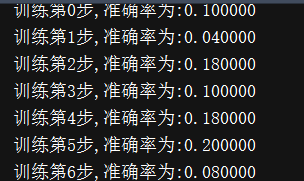2.书中教材案例（jupyter）

import tensorflow as tf
import tensorflow.examples.tutorials.mnist.input_data as input_data

#建立共享函数
def weight(shape):
return tf.Variable(tf.truncated_normal(shape, stddev=0.1),
name ='W')#返回截断类型的随机值，连个方差之间的随机值

def bias(shape):
return tf.Variable(tf.constant(0.1, shape=shape)#初值为0.1
, name = 'b')

def conv2d(x, W):
return tf.nn.conv2d(x, W, strides=[1,1,1,1],

def max_pool_2x2(x):
return tf.nn.max_pool(x, ksize=[1,2,2,1],
strides=[1,2,2,1],

#数据输入占位符
with tf.name_scope('Input_Layer'):
x = tf.placeholder("float",shape=[None, 784]
,name="x")
x_image = tf.reshape(x, [-1, 28, 28, 1])#卷积运算分维度，必须分开

#卷积1
with tf.name_scope('C1_Conv'):
W1 = weight([5,5,1,16]) #窗口大小5*5，厚度为1， 数量为16
b1 = bias()#对16个卷积窗口进行偏置赋值
Conv1=conv2d(x_image, W1)+ b1
C1_Conv = tf.nn.relu(Conv1 )#非线性化
with tf.name_scope('C1_Pool'):
C1_Pool = max_pool_2x2(C1_Conv)#特征压缩14*14

#卷积2
with tf.name_scope('C2_Conv'):
W2 = weight([5,5,16,36])#窗口大小为5*5， 接上一层厚度为16， 数量为36
b2 = bias()
Conv2=conv2d(C1_Pool, W2)+ b2
C2_Conv = tf.nn.relu(Conv2)
with tf.name_scope('C2_Pool'):
C2_Pool = max_pool_2x2(C2_Conv) #7*7

#全连接
with tf.name_scope('D_Flat'):
D_Flat = tf.reshape(C2_Pool, [-1, 1764])#展开进行全连接
with tf.name_scope('D_Hidden_Layer'):
W3= weight([1764, 128])#加一个全连接隐藏层
b3= bias()
D_Hidden = tf.nn.relu(
tf.matmul(D_Flat, W3)+b3)
D_Hidden_Dropout= tf.nn.dropout(D_Hidden,
keep_prob=0.8)

#输出层
with tf.name_scope('Output_Layer'):
W4 = weight([128,10])
b4 = bias()
y_predict= tf.nn.softmax(
tf.matmul(D_Hidden_Dropout,
W4)+b4)

#模型优化步骤
with tf.name_scope("optimizer"):

y_label = tf.placeholder("float", shape=[None, 10],
name="y_label")

loss_function = tf.reduce_mean(
tf.nn.softmax_cross_entropy_with_logits
(logits=y_predict ,
labels=y_label))

.minimize(loss_function)

#模型评估
with tf.name_scope("evaluate_model"):
correct_prediction = tf.equal(tf.argmax(y_predict, 1),
tf.argmax(y_label, 1))
accuracy = tf.reduce_mean(tf.cast(correct_prediction, "float"))

#训练
trainEpochs = 10
batchSize = 100
# totalBatchs = int(mnist.train.num_examples/batchSize)#将全部数据拿来进行训练，并且进行10遍，所以时间较长
totalBatchs = int(5)#时间太长了，所以只取前500个数据进行循环10次的训练
epoch_list=[];accuracy_list=[];loss_list=[];
from time import time #导入时间模块进行时间统计
startTime=time()
sess = tf.Session()#创建会话
sess.run(tf.global_variables_initializer())#初始化变量

for epoch in range(trainEpochs):

for i in range(totalBatchs):
batch_x, batch_y = mnist.train.next_batch(batchSize)
sess.run(optimizer,feed_dict={x: batch_x,
y_label: batch_y})

loss,acc = sess.run([loss_function,accuracy],
feed_dict={x: mnist.validation.images,
y_label: mnist.validation.labels})

epoch_list.append(epoch)
loss_list.append(loss);accuracy_list.append(acc)

print("Train Epoch:", '%02d' % (epoch+1), \
"Loss=","{:.9f}".format(loss)," Accuracy=",acc)

duration =time()-startTime
print("Train Finished takes:",duration)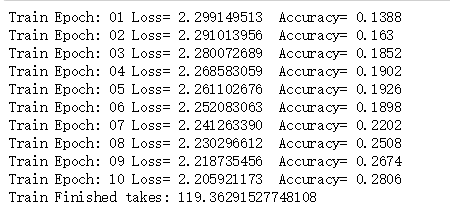• 显示准确率和损失值变化
• 准确率
plt.plot(epoch_list, accuracy_list,label="accuracy" )
fig = plt.gcf()
fig.set_size_inches(4,2)
# plt.ylim(0.8, 1) #因为训练数据太少所以准确度没有达到0.8以上所以一开始没哟显示
plt.ylim(0,1)#设置只查看的范围
plt.ylabel('accuracy')
plt.xlabel('epoch')
plt.legend()
plt.show()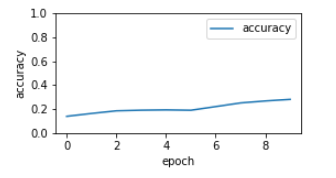* 损失值

%matplotlib inline
import matplotlib.pyplot as plt
fig = plt.gcf()
fig.set_size_inches(4,2)
plt.plot(epoch_list, loss_list, label = 'loss')
plt.ylabel('loss')
plt.xlabel('epoch')
plt.legend(['loss'], loc='upper left')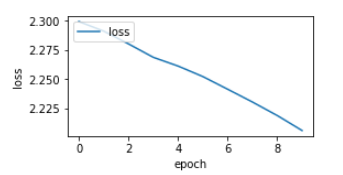• 检测模型准确率（加大数据量便可以提高）
print("Accuracy:",
sess.run(accuracy,feed_dict={x: mnist.test.images,
y_label: mnist.test.labels}))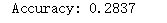• 查看softmax输出的概率预测
y_predict=sess.run(y_predict,
feed_dict={x: mnist.test.images[:5000]})#前5000个样本
y_predict[:5]#查看前五个样本对于10种分类分别得概率是多少

• 图像化的预测结果展示
prediction_result=sess.run(tf.argmax(y_predict,1),
feed_dict={x: mnist.test.images ,
y_label: mnist.test.labels})

#建立展示函数
import numpy as np
def show_images_labels_predict(images,labels,prediction_result):
fig = plt.gcf()
fig.set_size_inches(8, 10)
for i in range(0, 10):
ax=plt.subplot(5,5, 1+i)
ax.imshow(np.reshape(images[i],(28, 28)),
cmap='binary')
ax.set_title("label=" +str(np.argmax(labels[i]))+
",predict="+str(prediction_result[i])
,fontsize=9)
plt.show()
show_images_labels_predict(mnist.test.images,mnist.test.labels,prediction_result)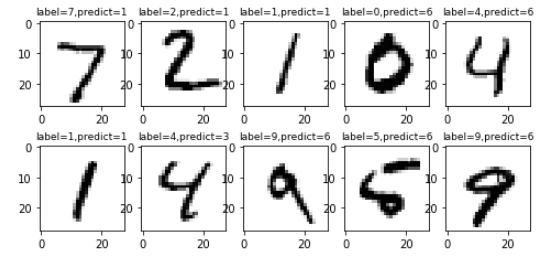• 选出识别错误的样本
for i in range(500):
if prediction_result[i]!=np.argmax(mnist.test.labels[i]):
print("i="+str(i)+
"   label=",np.argmax(mnist.test.labels[i]),
"predict=",prediction_result[i])

#将识别错误的样本进行展示
def show_images_labels_predict_error(images,labels,prediction_result):
fig = plt.gcf()
fig.set_size_inches(8, 10)
i=0;j=0
while i<10:
if prediction_result[j]!=np.argmax(labels[j]):
ax=plt.subplot(5,5, 1+i)
ax.imshow(np.reshape(images[j],(28, 28)),
cmap='binary')
ax.set_title("j="+str(j)+
",l=" +str(np.argmax(labels[j]))+
",p="+str(prediction_result[j])
,fontsize=9)
i=i+1
j=j+1
plt.show()
show_images_labels_predict_error(mnist.test.images,mnist.test.labels,prediction_result)#代表样本索引，真实值，预测值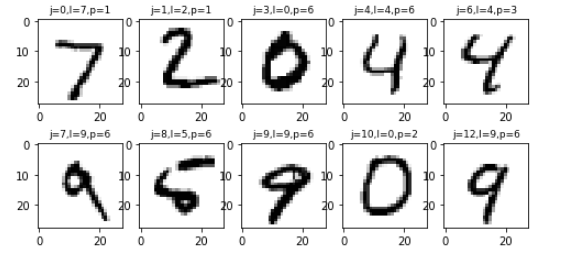展开全文• 本代码使用python基于TensorFlow设计手写数字识别算法，并编程实现GUI界面，构建手写数字识别系统。这是本人的本科毕业论文课题，当然，这个也是机器学习的基本问题。本博文不会以论文的形式展现，而是以编程实战...
• 本文使用python基于TensorFlow设计手写数字识别算法，并编程实现GUI界面，构建手写数字识别系统。这是本人的本科毕业论文课题，当然，这个也是机器学习的基本问题。本博文不会以论文的形式展现，而是以编程实战完成...
• tensorflow手写数字识别 python 编写qt界面演示视频：https://www.bilibili.com/video/BV1uz4y1r7P1?from=search&seid=9468972372585662716
• 下载后解压到指定文件夹即可，里面的4个压缩文件无需解压。
• 刚接触神经网络的可以参考参考，网络结构是全连接的，代码流程也比较清晰，用的MNIST数据集，运行起来也很快。
• 上一篇文章完成了简陋版的tensorflow手写数字实现（tensorflow自主学习入门-从零开始篇（二）tensorflow手写数字识别），虽然简陋，但是总的能从中学到一些东西，现在又赶紧将自己前一段时间学习到的关于tensorflow...python tensorflow 机器学习 人工智能
• 使用Python+TensorFlow，全连接的神经网络，基于MNIST数据集。数据集包含60000张训练图片，10000张测试图片。MNIST_model文件夹是已经训练30000次的模型，也可以自己再训练。app.py文件可以测试自己的图片。 也可以...
• 基于python，tensorflow实现的关于手写数字识别。适于初学者python
• python语言编写，利用TensorFlow建立两层卷积神经网络，数据集为手写识别数据集MNIST，识别准确率99%
• 由于pip安装的TensorFlow缺少一部分代码，以及TensorFlow2版本相对于TensorFlow1在语句上有变化。因此大部分网上代码不适用（主要问题在于读取mnist数据包和一些函数形式有问题）。这个代码可以运行。内含离线mnist...
• tensorflow实现手写数字识别

千次阅读 2021-10-19 18:25:45
手写数字识别 目录过程1.导入库及获取数据集2. 整理数据集3.定义各种参数及方法4.神经网络训练5.可视化损失和准确率6.预测及可视化完整代码 过程 1.导入库及获取数据集 这里采用Keras中常用数据集Datasets中的...tensorflow python 深度学习
• 主要为大家详细介绍了基于TensorFlow的CNN实现Mnist手写数字识别，文中示例代码介绍的非常详细，具有一定的参考价值，感兴趣的小伙伴们可以参考一下
• 基于tensorflow手写数字识别程序代码。从获取mnist数据库开始到最后的运行精度显示。这个是基于mnist的数据库进行训练和识别的
• 基于TENSORFLOW的手写数字识别

千次阅读 2020-07-02 18:45:46
Tensorflow框架为深度学习工具的应用已经相当广泛，卷积神经网络是一类包含卷积运算且具有深度结构的前馈神经网络，采用反向传播(Back Propagation,BP)算法对模型进行学习训练，手写字体识别模型LeNet5诞生于1994...机器学习 神经网络 python tensorflow
• 主要为大家详细介绍了基于Tensorflow的MNIST手写数字识别分类，文中示例代码介绍的非常详细，具有一定的参考价值，感兴趣的小伙伴们可以参考一下
• 手写数字图片数字集       机器学习需要从数据中间学习，因此首先需要采集大量的真实样本数据。以手写的数字图片识别为例，我们需要收集大量的由真人书写的0-9的数字图片，为了便于......